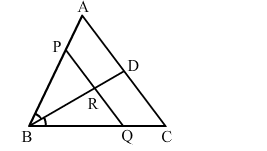# In ∆ABC, the bisector of ∠B meets AC at D. A line PQ ∥ AC meets AB, BC and BD at P,Question:

In ∆ABC, the bisector of ∠B meets AC at D. A line PQ ∥ AC meets ABBC and BD at P, Q and R respectively.
Show that PR × BQ = QR × BP.Solution:

In triangle ​BQP, BR bisects angle B.
Applying angle bisector theorem, we get:

$\frac{Q R}{P R}=\frac{B Q}{B P}$

$\Rightarrow B P \times Q R=B Q \times P R$

This completes the proof.